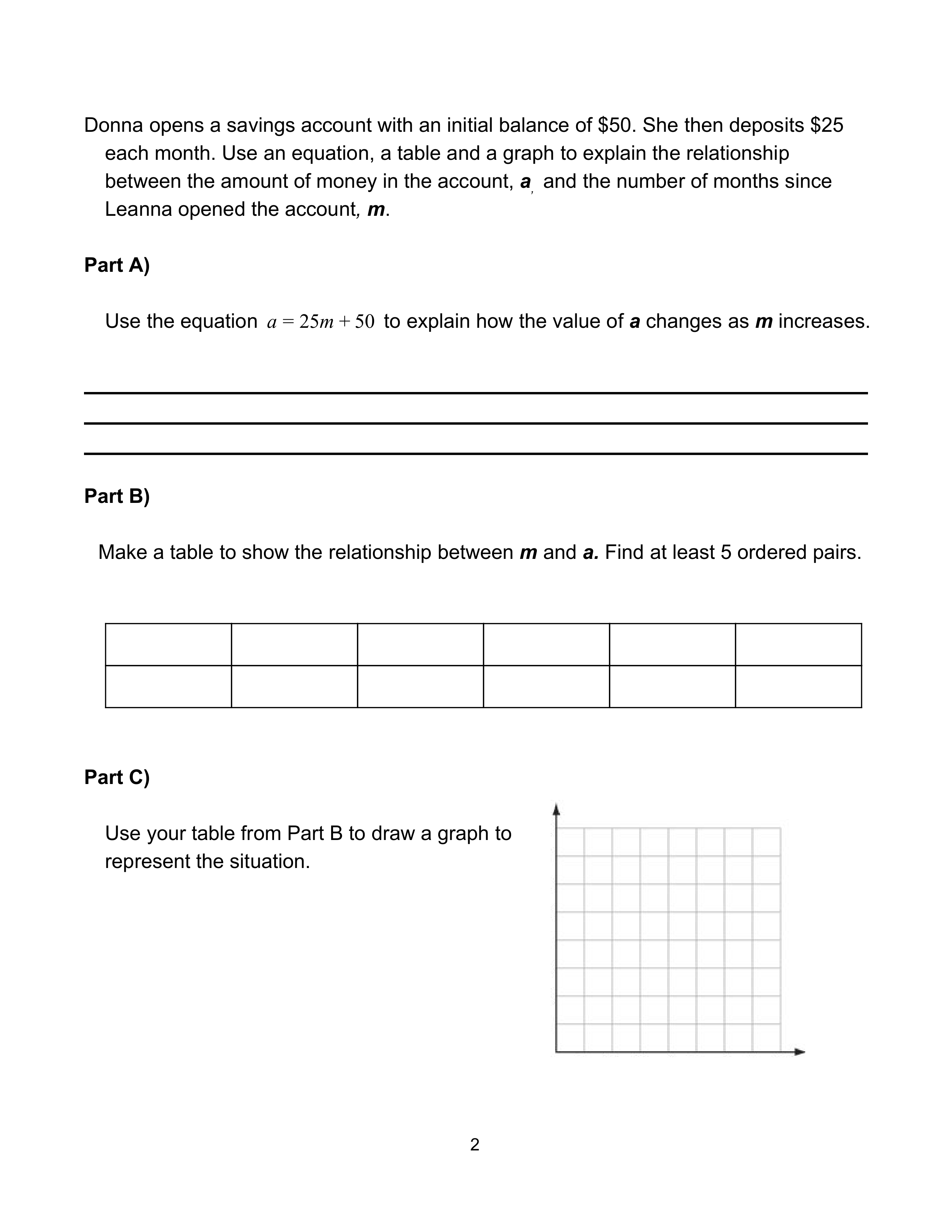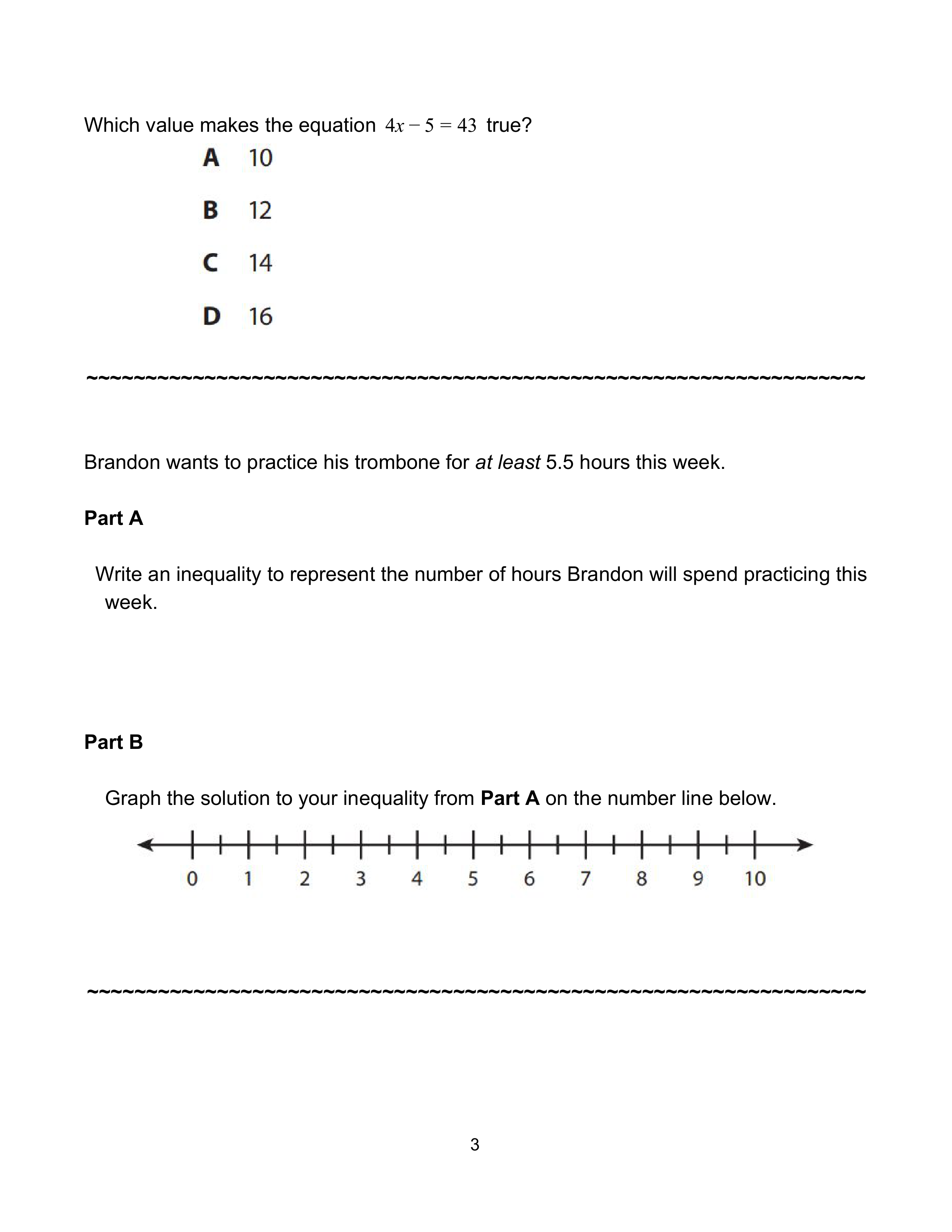Unit 3 Test Form B - Retake
starstarstarstarstarstarstarstarstarstar
by Christine Godfrey
| 21 Questions
Note from the author:
Ready Math - Expression and Equationsa
1
c
d
2
f
3
h
4
play_arrow
1
1
What is the constant in the following expression?

A
B
C
D
play_arrow
A company sells speakers for \$23 each. At the end of the week, they have sold \$851 worth of speakers. How many speakers did they sell? Write and solve an equation to find the answer.
2
1
Write an equation to represent the situation. Use the variable x.
play_arrow
3
1
The company sold _____ speakers.
play_arrow
4
2.5
If each equation below was solved for x, for which equation is x = 6 the solution?
Choose all that apply.
A
B
C
D
Ea
b
c
5
e
6
g
7
play_arrow
Donna opens a savings account with an initial balance of \$50. She then deposits \$25 each month. Use an equation, a table and a graph to explain the relationship between the amount of money in the account, a, and the number of months since Donna opened the account, m.
play_arrow
5
1
Part A)
Use the equation a=25m+50 to explain how the value of a changes as m increases.

play_arrow
6
3
Part B)
Make a table to show the relationship between m and a. Find at least 5 ordered pairs. Given the equation a = 25m + 50.
play_arrow
7
4.5
Use your table from Part B to draw a graph to represent the situation.a
8
c
d
e
9
g
10
play_arrow
8
1
Which value makes the equation 4x - 5 = 43 true?
A
B
C
D
play_arrow

# Brandon wants to practice his trombone for at least 5.5 hours this week.

play_arrow
9
1
Part A
Write an inequality to represent the number of hours Brandon will spend practicing this week. Use the variable x.
play_arrow
10
3
Part B
Graph the solution to your inequality from Part A on the number line below.a
11
c
d
12
13
14
15
16
j
17
play_arrow
11
2.5
Which expression is equivalent to 45x - 15x?
Choose all that apply.
A
B
C
D
E
play_arrow
Consider the possible solution to each inequality. Choose Yes or No for each question.
12
0.5
Is 8.3 a solution of

Yes
No
13
0.5
Is 6.5 a solution of

Yes
No
14
0.5
IS 7 a solution of

Yes
No
15
0.5
Is 17 a solution of

Yes
No
16
0.5
Is 6 a solution of

Yes
No
play_arrow
17
2
Evaluate:a
b
c
18
e
19
g
20
i
21
play_arrow
Jen accidently knocks a square framed poster off the wall, breaking the protective glass piece. The glass piece has side length x and she needs to buy a new piece of glass to replace the it.
play_arrow
18
1
Part A
Write a variable expression for the area of the glass piece Jen needs to replace.
play_arrow
19
1
Part B
If the protective glass piece is 25 inches wide, what is the area of the glass piece Jen needs to replace?
play_arrow
20
1
Part C
If Jen has a budget of \$150, what is the most she can pay per square inch of glass to replace the glass piece described in Part B?
play_arrow
21
1